﻿ Math Icon Set 2 stock vector. Illustration of symbol, subtract - 5451881

# Math Icon Set.

## 16 icon set of mathematical operations symbols

Designers Also Selected These Stock Illustrations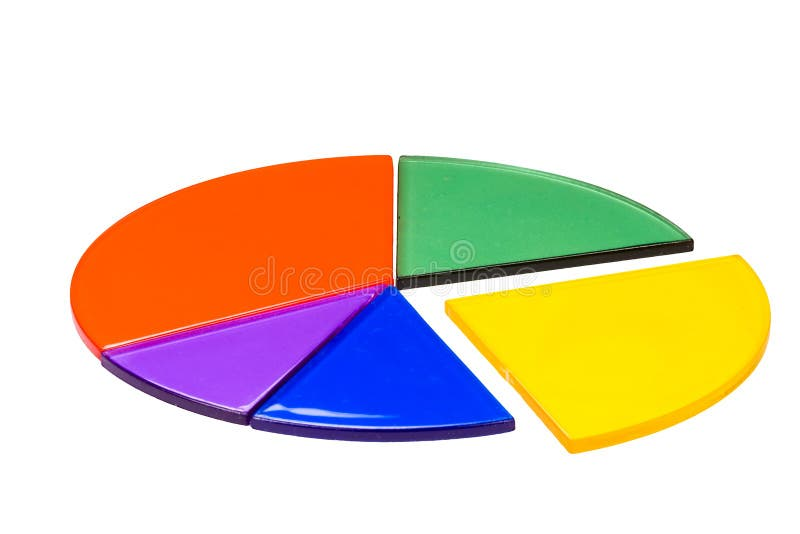Isolated circles graph made from fraction circles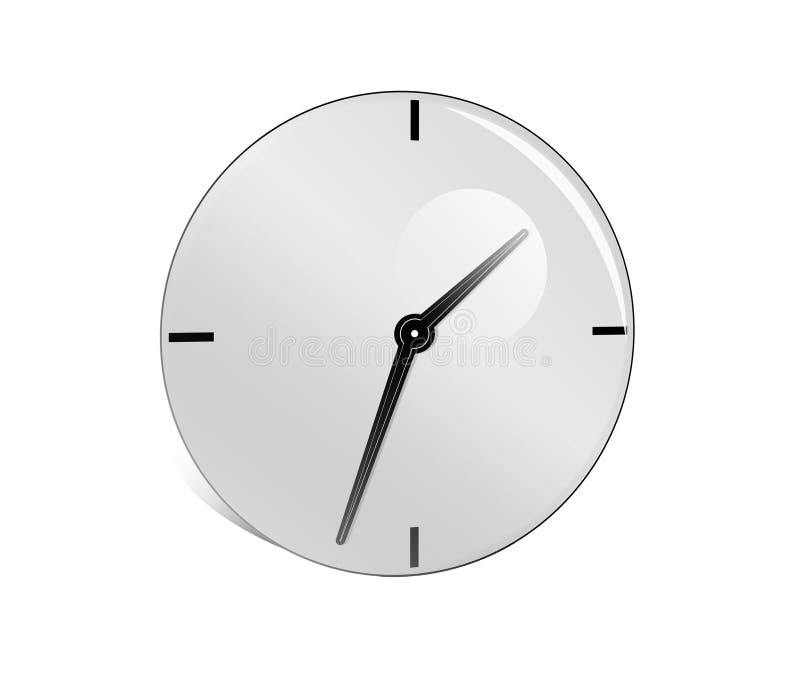Clock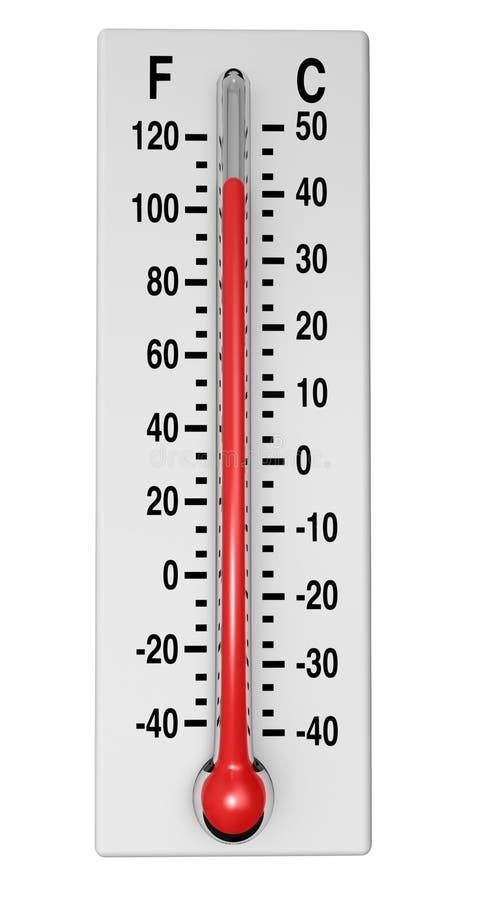Classic Thermometer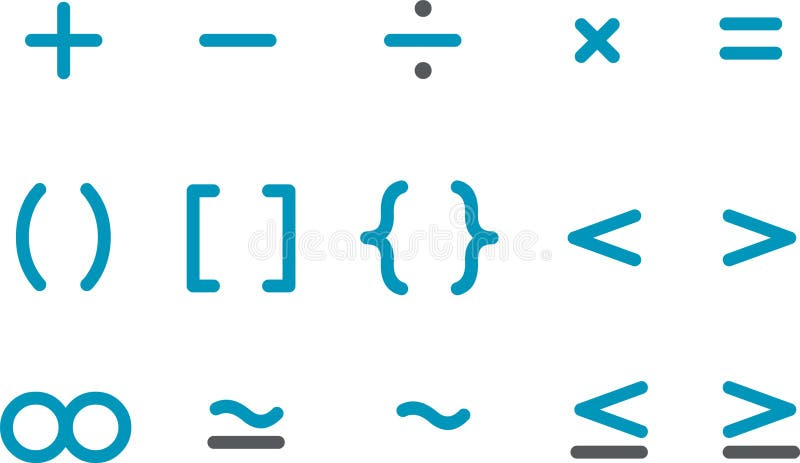Math Icon SetHuman heart red backgroundScience, Math, Art and Music Bubble Boy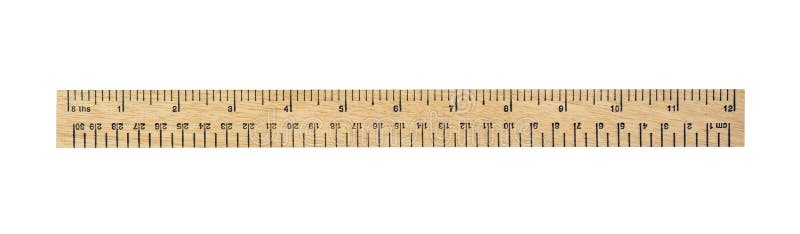Ruler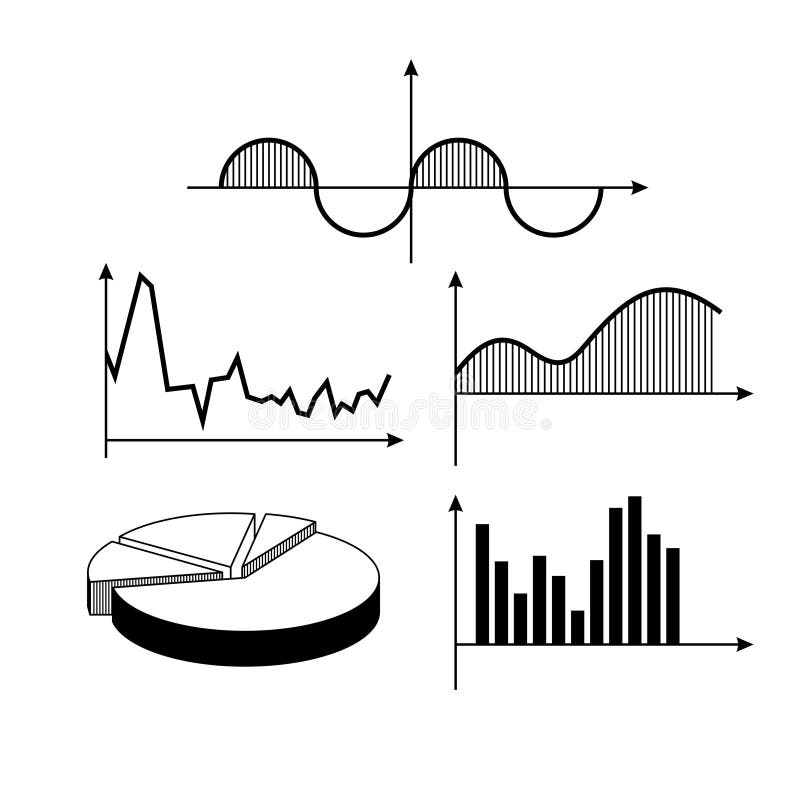Graph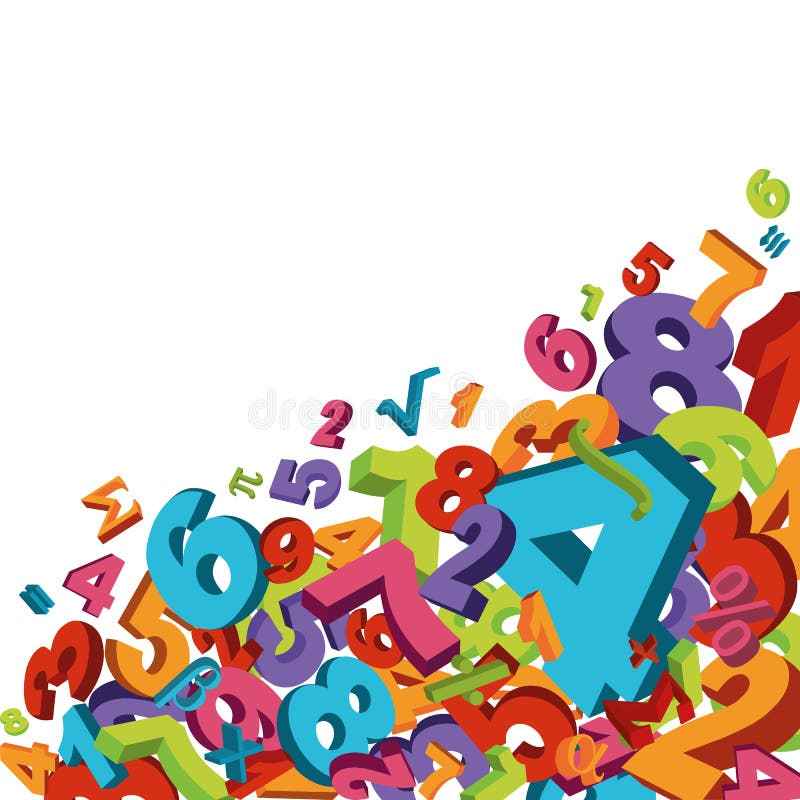Mathematical background
More similar stock illustrations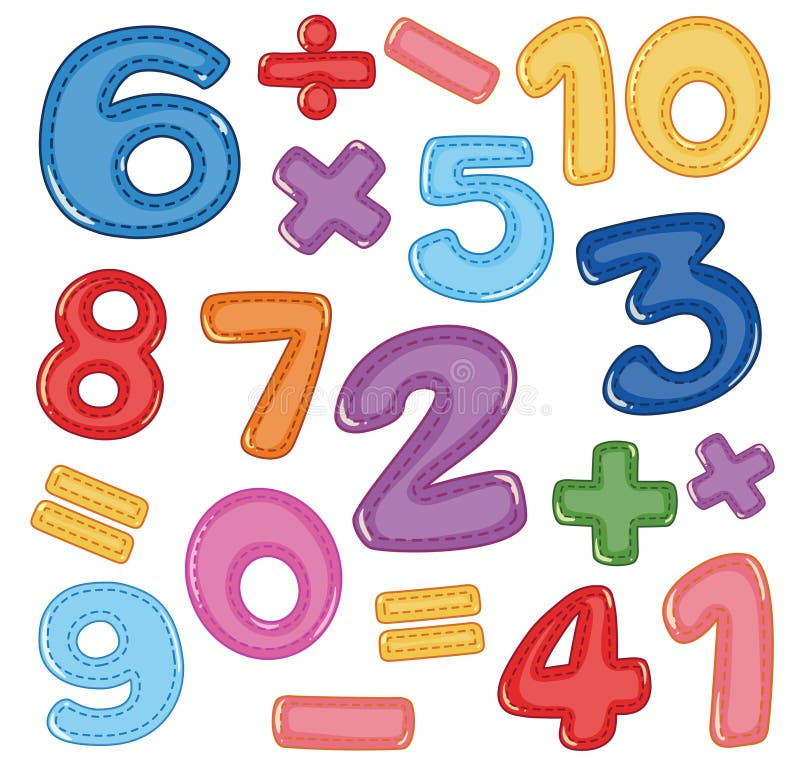A set of number and math icon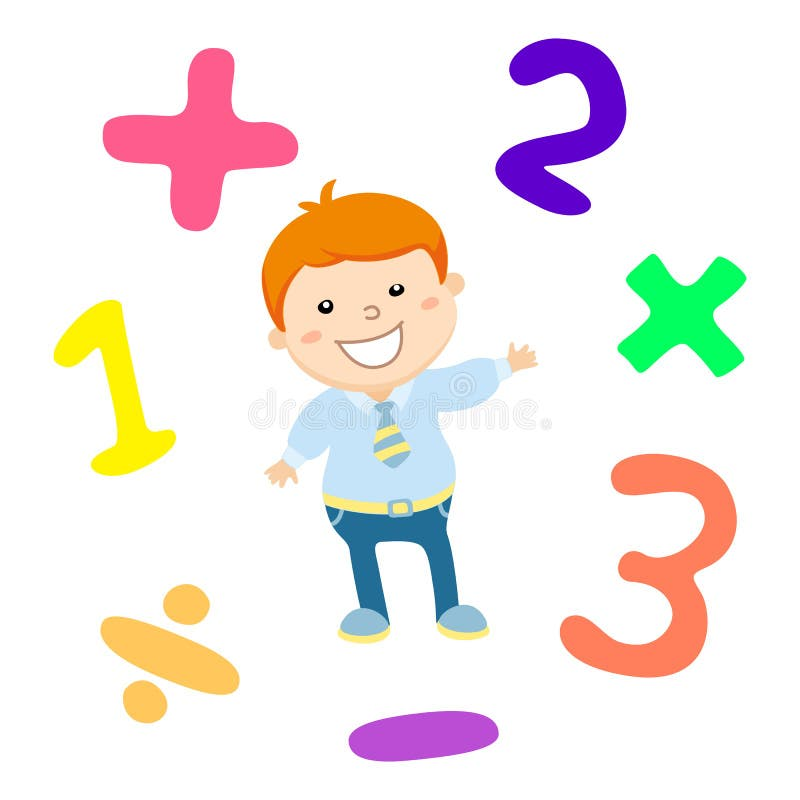Cartoon style math learning game illustration. Mathematical arithmetic logic operator symbols icon set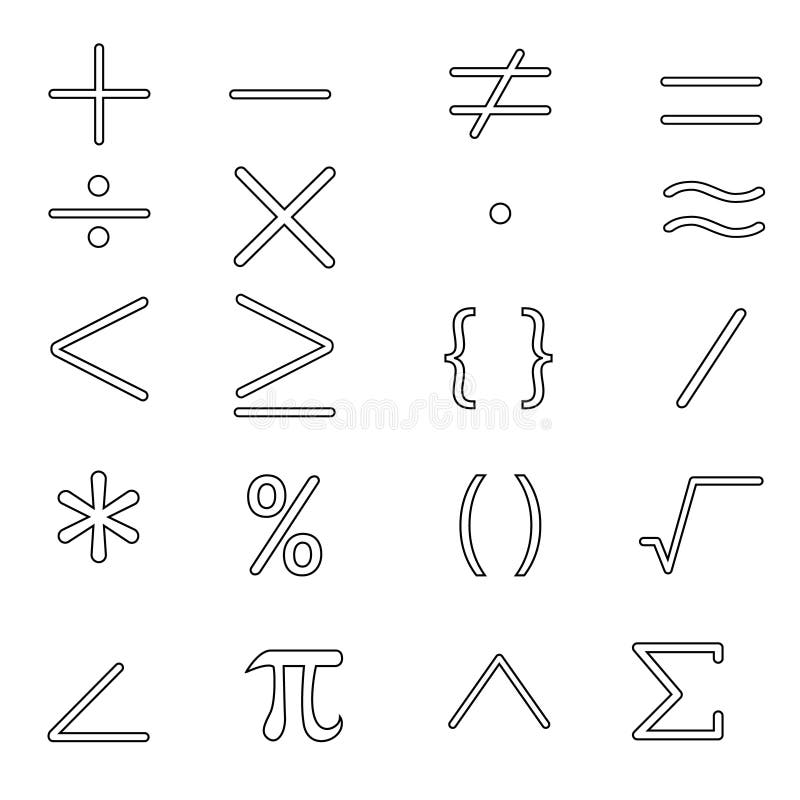Math icon vector set. mathematical calculations symbol illustration collection.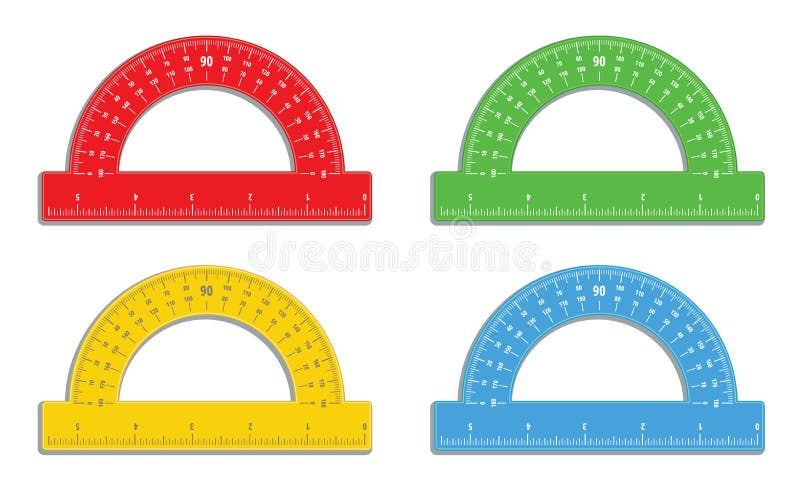Set of realistic colorful protractors with 6 inch ruler icon. Math measure tool. Instrument for measuring angles. School supplies.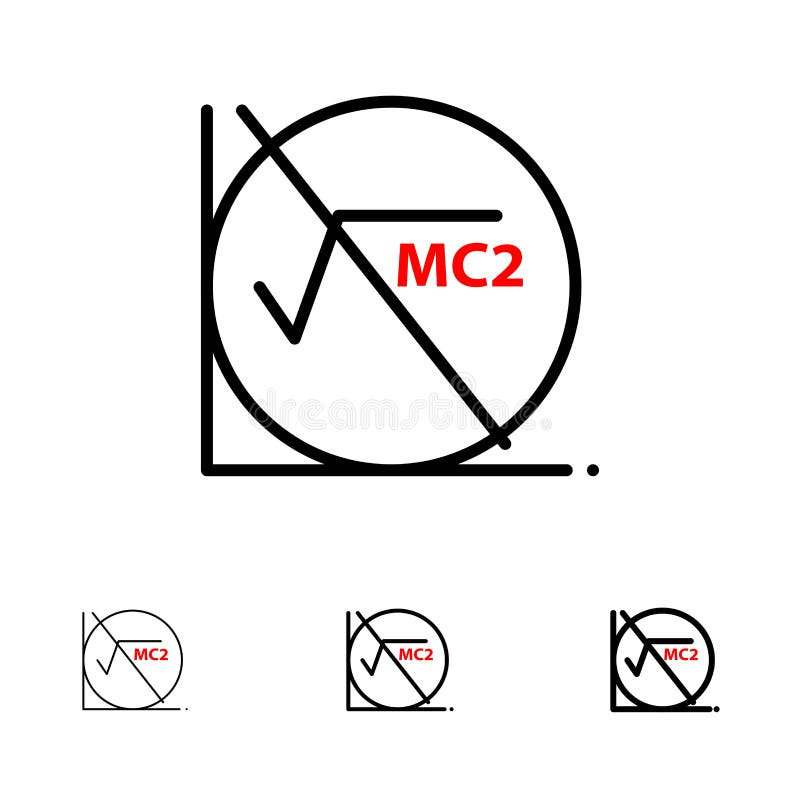Math, Formula, Math Formula, Education Bold and thin black line icon set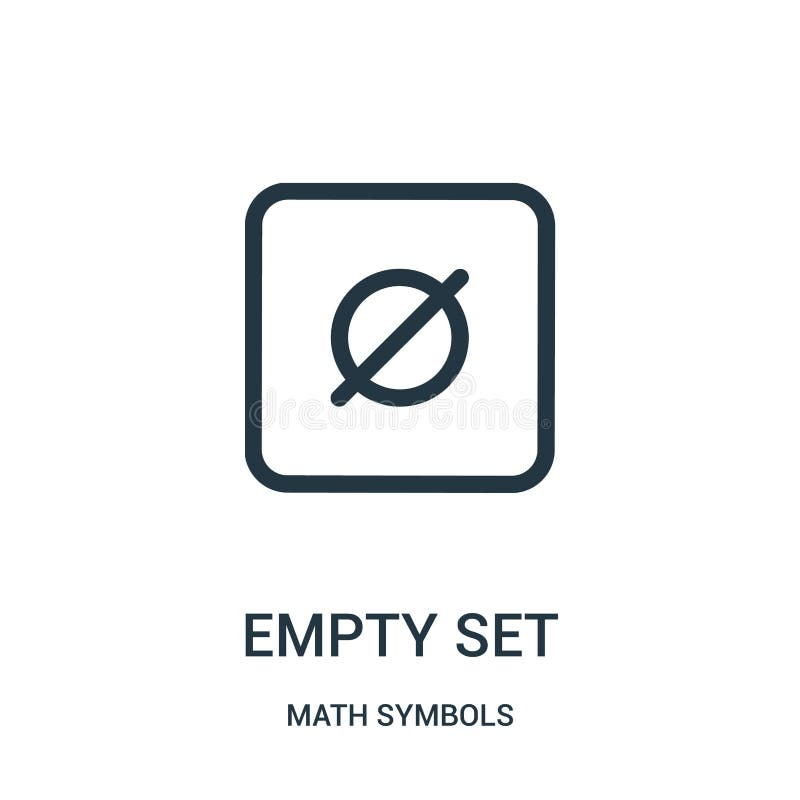empty set icon vector from math symbols collection. Thin line empty set outline icon vector illustration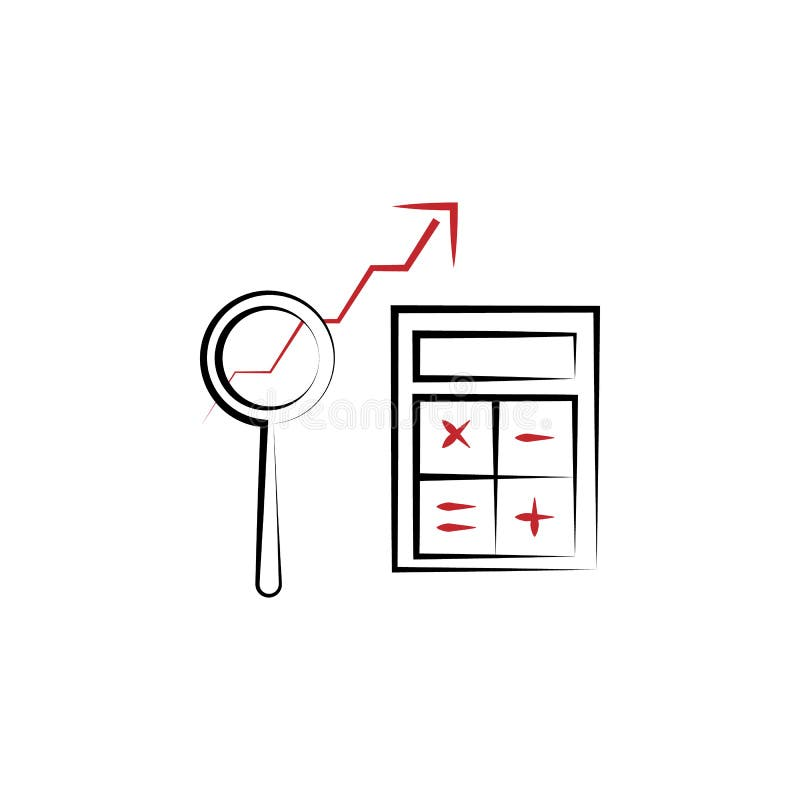math's 2 colored line icon. Simple colored element illustration. math's outline symbol design from edecutaion set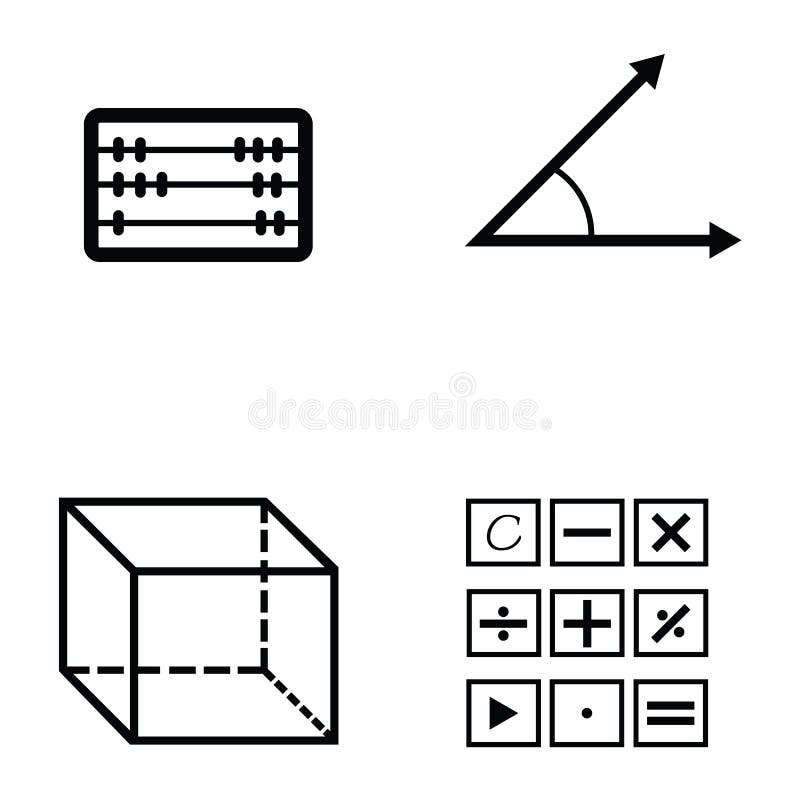Math icon set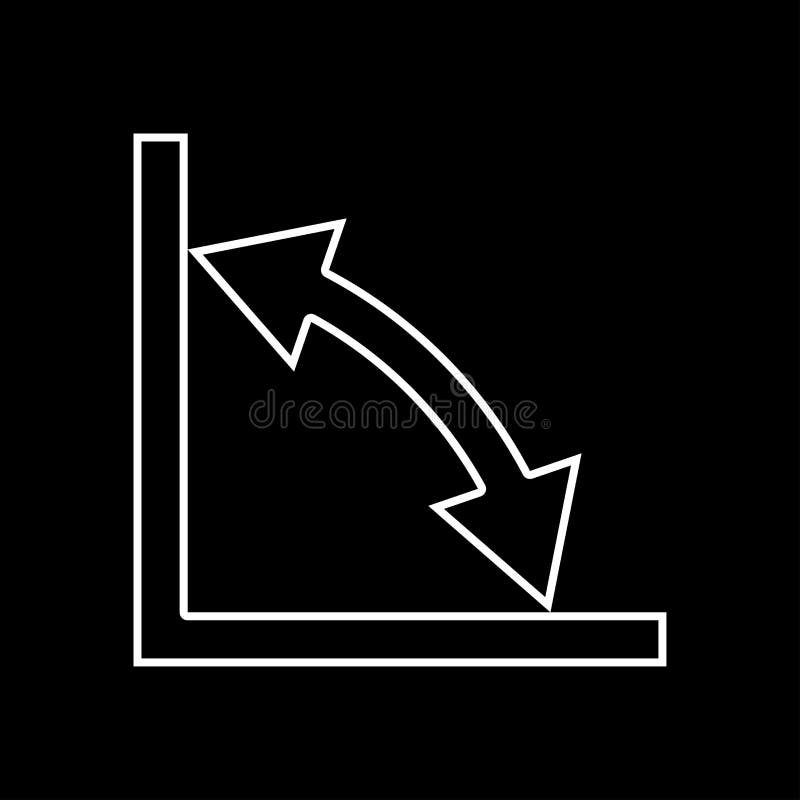Geometry math signs symbols grey set icon .
More stock photos from Brunoil's portfolio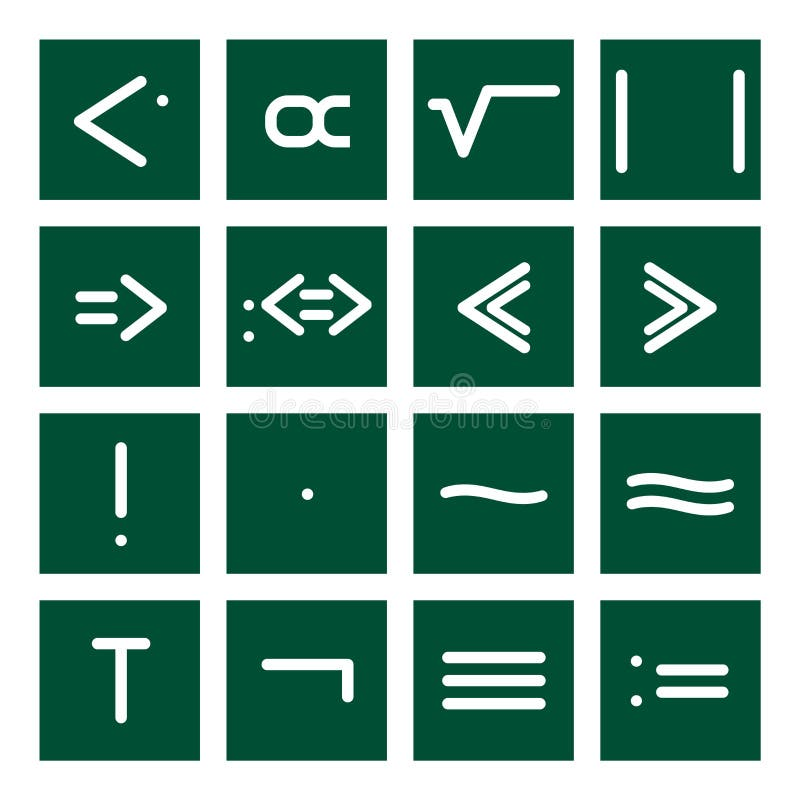Math Icon Set 4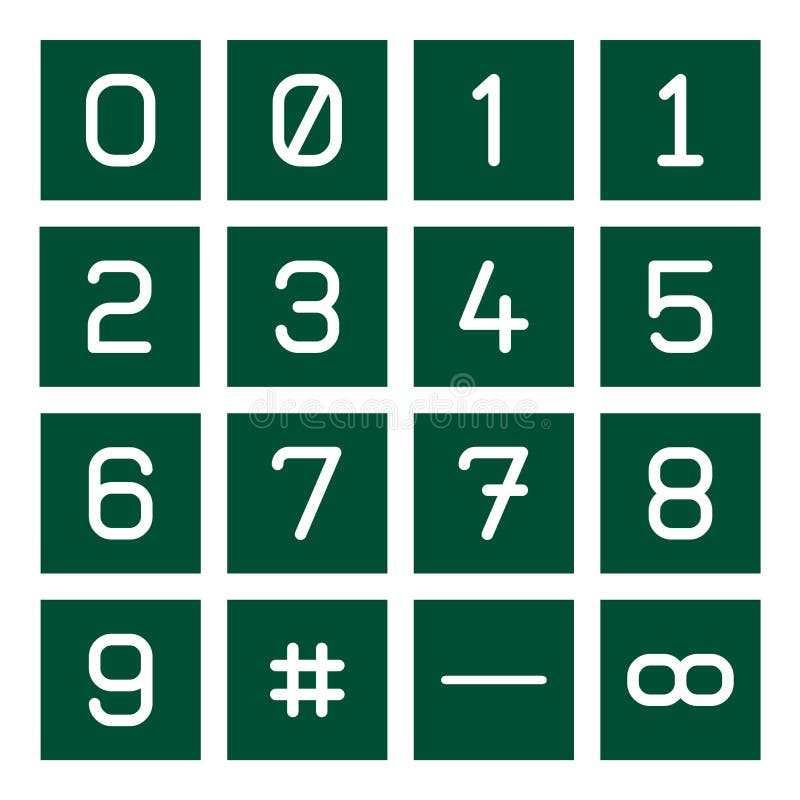Math Icon Set 1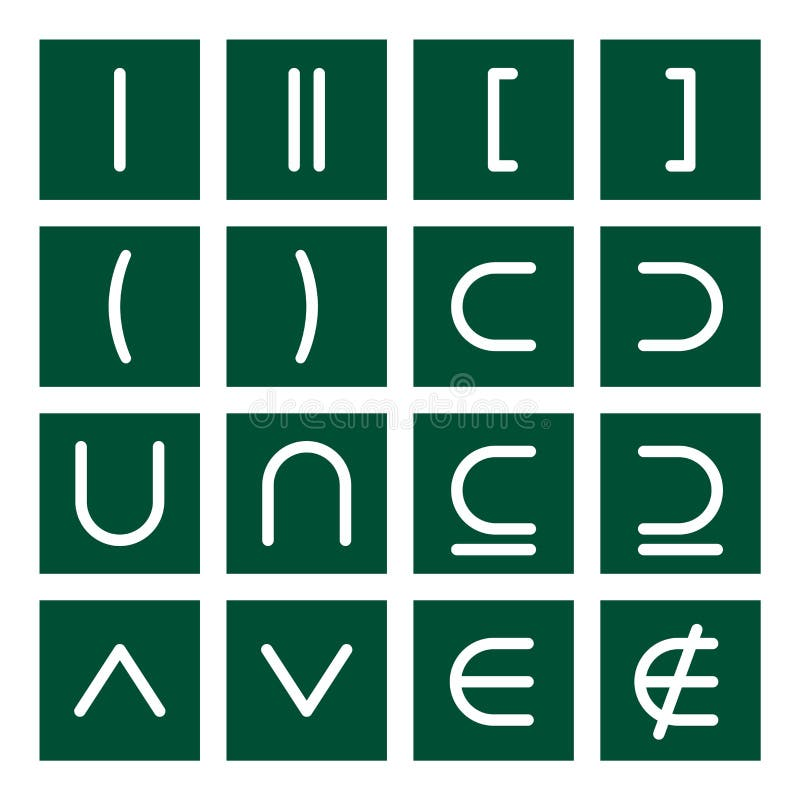Math Icon Set 3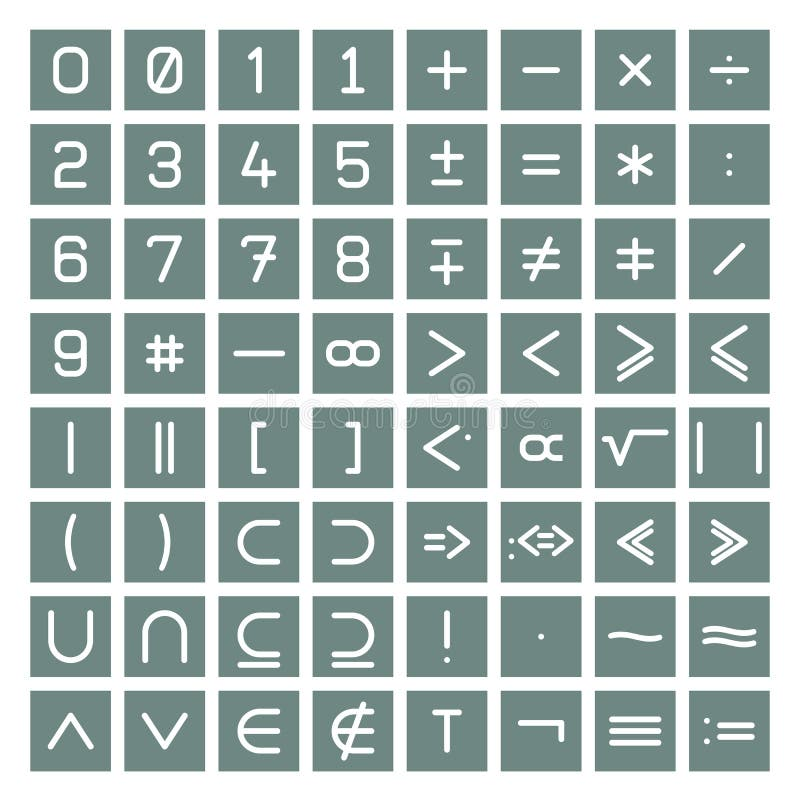Mathematics Symbols Collection
Related categories